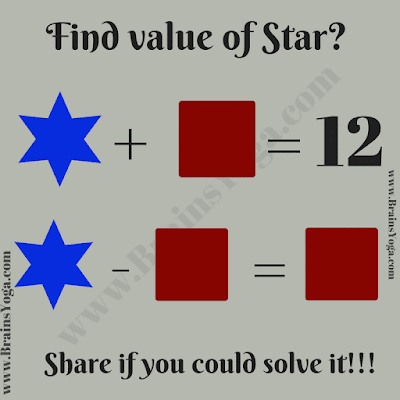Here is simple Mathematical Equation Picture Puzzle for kids. Can you solve these two equations and find the value of both Square and Star?Can you find value of Star and Square?